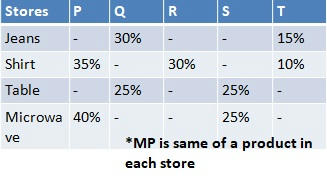# Data Interpretation (Set-63) | Maths & Reasoning | Free PDF Download

1.The percentage discount on Jeans at store R is 1.5 times that of the percentage discount on Jeans at store Q. If the difference between selling price of Jeans at stores T and S together and selling price of Jeans at stores R and S together is Rs. 3000, then what is the marked price of Jeans?a) Rs 30000    b) Rs 13000 c) Rs 9000 d) RS 20000 e) Rs 10000

2.Total discount on Microwave at stores P and Q together is Rs.30000 and total discount on Microwave at stores Q and S together is Rs.24000. If percentage discount on Microwave in store Q is 20 percentage point more than the percentage discount on Microwave in store R, then what is the average selling price of Microwave at stores Q and R together?
a) Rs 25000 b) Rs 30000 c) Rs 36000 d) Rs 35000 e) Rs 40000

3.The percentage discount on table at store R is 60% more than that of at store Q and the percentage discount on table at store P is 25% less that of at store R. If the profit on table at store S is 12.5%, then what is the average percentage profit/loss on table at stores P and R together?
a) profit 2%    b) profit 3%     c) profit 4%    d) profit 5%      e) Loss 2.5%

4.The percentage discount on Microwave at store T is 60% of percentage discount on table at store Q and the percentage discount on Microwave at store Q is 5 more than twice the percentage discount on Jeans at store T. If the profit on Microwave at store T is Rs 8000 more than the profit on Microwave at store Q, then what is the marked price of Microwave?
a) Rs 30000 b) Rs 35000 c) Rs 40000 d) Rs 45000 e) Rs 50000

5.The total discount on Shirt at stores P and S together is Rs 400 more than the total discount on Shirt at stores P and Q together. If the percentage discounts on Shirt at store Q is 5 percentage point less than the percentage discounts on Shirt at store S and selling price of Shirt at stores P and S together is Rs.11600, then what is the total discount on Shirt at stores Q and S together?
a) 1700      b) 1600     c) 2800     d) 3000     e) None